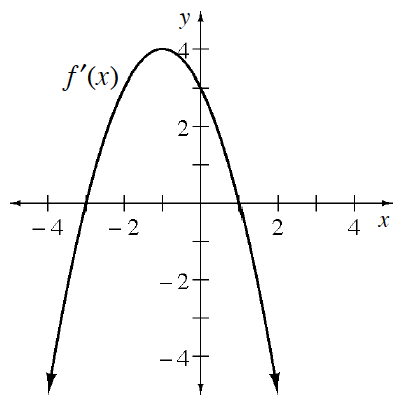### Home > APCALC > Chapter 5 > Lesson 5.2.4 > Problem5-98

5-98.

Using the graph of $y=f^\prime(x)$ below, determine the values of $x$ where $f$ has a local minimum, local maximum, or point of inflection. Justify your answer for each point.You are looking at a graph of $f^\prime\left(x\right)$, which shows all the slopes of $f\left(x\right)$.

A local minimum on $f\left(x\right)$ is located where the slopes of $f\left(x\right)$ change from negative to positive.

A local maximum on $f\left(x\right)$ is located where the slopes of $f\left(x\right)$ change from positive to negative.

An inflection point on $f\left(x\right)$ is where concavity changes, and concavity is a determined by the slope of the the slopes.

$x = −3$: local minimum
$x = 1$: local maximum
$x = −1$: inflection point